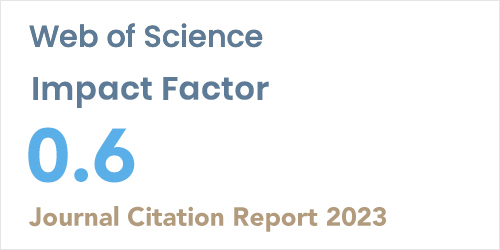ISSN: 1304-7191 | E-ISSN: 1304-7205
Volume: 41 Issue: 5 Year: 2023Calculation of buckling loads of ipe-section bending members based on optimization of analytical formulation
1Faculty of Engineering, Civil Engineering Department, Erciyes University, Kayseri, 38039, Turkey
2College of Engineering, Civil Engineering Department, University of Kirkuk, Kirkuk, 36001, Iraq
Sigma J Eng Nat Sci - DOI: 10.14744/sigma.2023.00031

#### Abstract

The critical lateral buckling load of cantilever beams with IPE cross-section was calculated using analytical closed-form equations and numerical finite element analyses within the scope of the research. The equations suggested in the specifications for simply supported beams were used to calculate the buckling load of cantilever beams. The rationality of the values calculated due to this is not fully known. In the research, a single loading was made to the shear center at the free end of the cantilever beam. Cantilever length and section height were kept variable. As a result, it has been determined that there are partial differences in the analysis result obtained from the elastic stability theory and finite element method. Accordingly, the results obtained from ANSYS and SAP2000 analyses confirm each other. On the other hand, the results obtained using the formulation of Timoshenko and Gere, the calculation results made according to the AISC and DCCPSS regulations, and the results obtained from the LTBeam program confirm each other. However, it differs from the FEA analysis due to the cantilever beam length's shortening and the section height increase. Thus, to obtain accurate and reliable results in the buckling load calculation of cantilever beams, the equations used in analytical calculations were optimized according to finite element analysis (FEA) results. As a result of the study conducted according to the error criteria, it was determined that the updated equation results gave similar results to the FEA results.